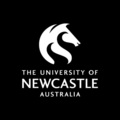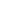Maths for Science is a Course

Maths for Science

Self-paced

Full course description

Galileo once said that "mathematics is the language of science", by which he meant that to understand and describe the natural world quantitatively requires the use of mathematics.

This course aims to introduce students to a range of mathematical concepts which are directly applicable in the sciences.

Topics covered include:

• Measurements: significant figures, scientific notation, units and the metric system
• Algebra: evaluating and rearranging formulas, proportionality
• Graphing: drawing graphs, graphing proportional and logarithmic relationships